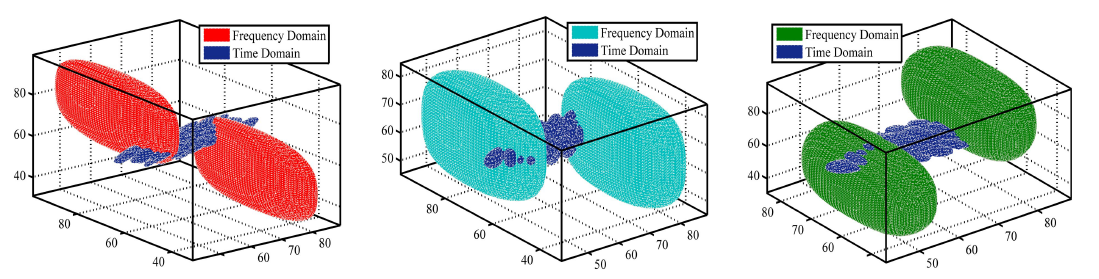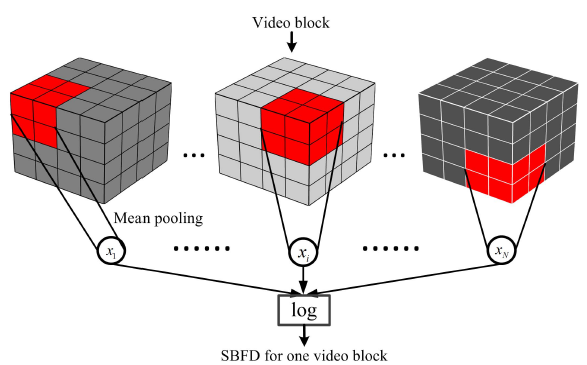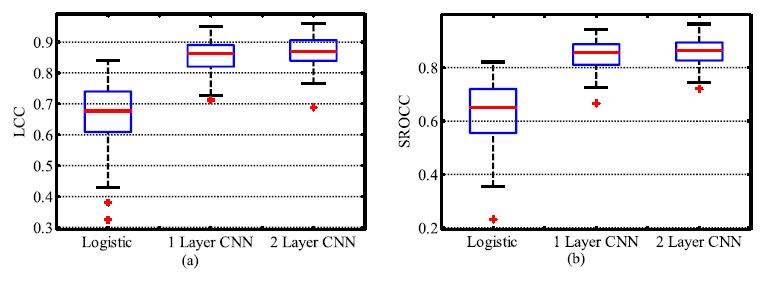# [Paper] SACONVA: Shearlet- and CNN-based NR VQA (Video Quality Assessment)

## Outperforms V-BLIINDS, SSIM & PSNR

In this story, No-Reference Video Quality Assessment With 3D Shearlet Transform and Convolutional Neural Networks (SACONVA), by City University of Hong Kong, and Chu Hai College of Higher Education, is briefly presented. This is a paper introduced by colleague when I study on IQA/VQA. In this paper:

• Taking video blocks as input, spatiotemporal features are extracted by 3D shearlet transform.
• Then, CNN and logistic regression are concatenated to predict a perceptual quality score.

This is a paper in 2016 TCSVT with over 50 citations where TCSVT has a high impact factor of 4.133. (Sik-Ho Tsang @ Medium)

# Outline

1. Spatiotemporal Feature Vector Formation
2. CNN and Logistic Regression
3. Experimental Results

# 1. Spatiotemporal Feature Vector Formation3D shearlets in the time domain and the frequency domain.
• (I’m not here to present the shearlet since shearlet transform is more likely in the field of signal processing which is another big topic. And I want to focus on neural network as usual. Nevertheless, we can treat it as a functional block to extract features from a multidimensional data.)
• In brief, the shearlet representation can to efficiently capture anisotropic features in multidimensional data.
• In terms of video block data, spatiotemporal features are captured.
• When the distortions are introduced into natural videos, the property of the distorted video will become different from the natural video.
• According to the differences, we can predict the quality score.Calculation process of SBFD for one video block
• The derivation of shearlet-based feature descriptors (SBFD) for one video block is as shown above.
• After 3D shearlet transform, mean (average) pooling is performed (red color), to get the values of x1 to xN.
• The pooled values are concatenated as a vector every element in this vector is subject to a logarithmic nonlinearity:
• The mean SBFD decreases as the amount of perceived distortion in the video increases.
• This feature vector is called primary SBFD. In the paper, the size of the vector is a 52 vector.

# 2. CNN and Logistic Regression

• After extracting the spatiotemporal features using 3D shearlet, CNN is used as the feature evolution process to evolve the primary SBFDs before being sent to the softmax classifier.
• Before being sent into the CNN, the input SBFD is normalized by subtracting the mean and dividing by the standard deviation of its elements.
• The proposed 1D CNN consists of five layers: Two fully connected convolutional layers, two max pooling layers and one output layer.
• “For CNN, the kernel number and kernel size are 10 and 19, respectively, for the first convolution layer, and 100 and 10, respectively, for the second convolution layer. The max-pooling size is two for each subsampling layer.” As directly quoted from the paper, I believe that 1D CNN is used, though it is mentioned as “fully connected”. (This might need to read the codes to verify.)
• The output layer is regressed to predict the score.
• There can be distortion type classification by applying softmax at the end, as shown above.
• The activation function for the intermediate layers used is sigmoid. (Right now, ReLU variants are more popular.)
• Convolutional autoencoder (CAE) and linear autoencoder (AE) are used to initialize the CNN. (At that moment, autoencoder is used to initialize weights for easier converge. Right now, random initialization is also possible.)

# 3. Experimental Results

## 3.1. Effects of Feature Evolution ProcessBox plot of LCC and SROCC on LIVE
• The primary SBFD is first sent into the logistic regression directly, and then CNN with one and two convolution layers are added between the SBFD and logistic regression. The quality estimation performance of these three situations.
• The performance is increased if the primary SBFD is evolved before sending into the logistic regression.

## 3.2. SOTA Comparison

• The performance of SACONVA outperforms the state-of-the-art NR-VQA method V-BLIINDS and is close to the FR-I/VQA methods.
• There are still other experiments to study the network size, distortion type classification, and also the experiments for the VQA applications. Please free feel to read the paper.

In this paper, handcrafted features are extracted first, then input into neural network for regression to predict the quality score.

## Video Quality Assessment

FR: [DeepVQUE]
NR: [SACONVA] [3D-CNN+LSTM]

--

--